(0) E′ → E
(1) E → E+T
(2) E → T
(3) T → T*F
(4) T → F
(5) F → (E)
(6) F → id

X FOLLOW( X )
E ), +, \$
T ), +, \$ , *
F ), +, \$ , *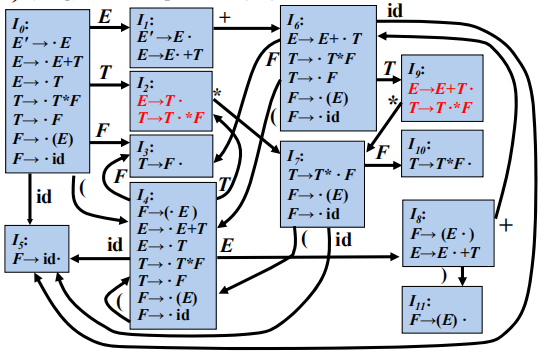### SLR 分析

SLR分析法的基本思想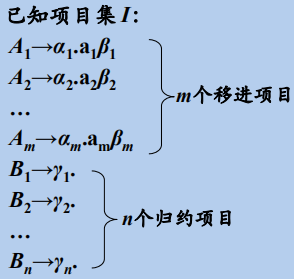• 若a∈{ a1, a2, …, am }，则移进a
• 若a∈FOLLOW(Bi)，则用产生式
Bi→γi 归约
• 此外，报错

### 表达式文法的SLR分析表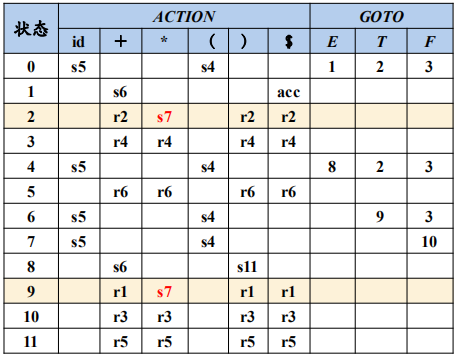(0) S′→ T
(1) T → aBd
(2) T → ε
(3) B →Tb
(4) B → ε

FOLLOW(S′ )={ \$}

FOLLOW(T) = {\$, b }

FOLLOW(B)={ d }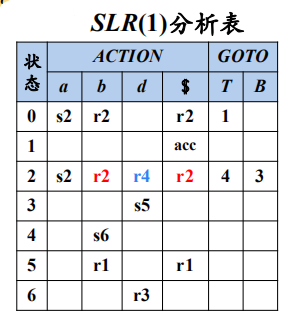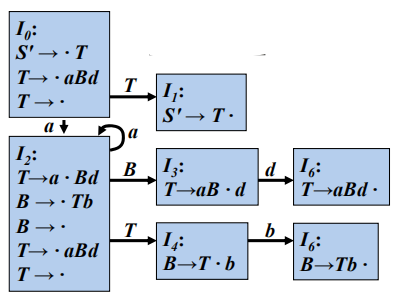### SLR 分析表构造算法

• 构造G'的规范LR(0)项集族C = { I0, I1, … , In}。

• 根据Ii构造得到状态i。状态i 的语法分析动作按照下面的方法决定：

• if A→α·aβ∈Ii and GOTO( Ii , a )=Ij then ACTION[ i, a ]=sj
• if A→α.Bβ∈Ii and GOTO( Ii , B )=Ij then GOTO[ i, B ]=j
• if A→α·∈Ii且A ≠ S' then for ∀a∈FOLLOW(A) do ACTION[ i, a ]=rj （j是产生式A→α的编号）
• if S'→S·∈Ii then ACTION [ i , \$ ]=acc;
• 没有定义的所有条目都设置为“error”。

### SLR 分析中的冲突

0) S′→S
1) S→L=R
2) S→R
3) L→*R
4) L→id
5) R→L

FOLLOW(R) = { =,\$ }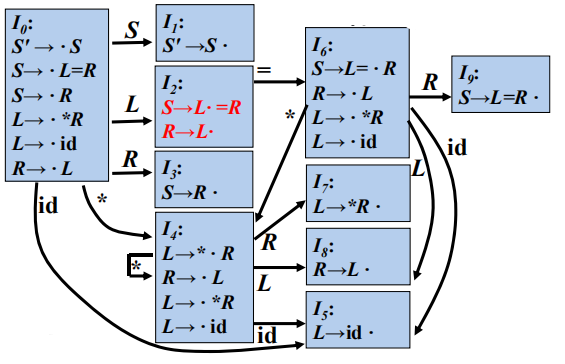原文链接: